# Quiz 1: Limits, Alternatives, and Choices

Business

(a) We can expect that when there is more rain, more umbrellas would be sold. This exhibits a direct relationship between the number of inches of rainfall in a month and the sale of umbrellas and can be represented by the graph as shown below.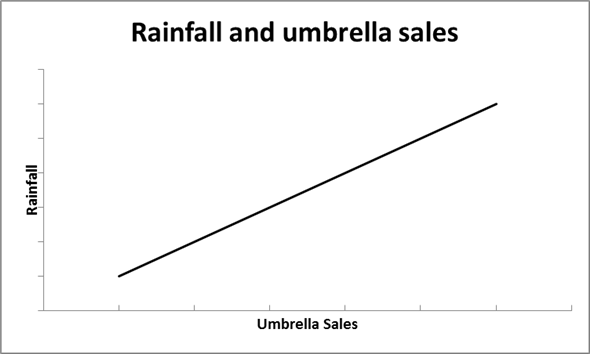(b) The amount of tuition and the level of enrollment at a university gives exaggerated examples. If tuition for a year at a university is one million dollars then, there are more chances that the enrollment will be zero, or extremely low. On the other hand, if a university's tuition is one hundred dollars a year then, there are chances that many people will go for enrollment at this university despite its quality. So, we can say that the higher the price of tuition, the lower the enrollment. These two variables exhibits an inverse relationship, and can be represented by the graph as shown below.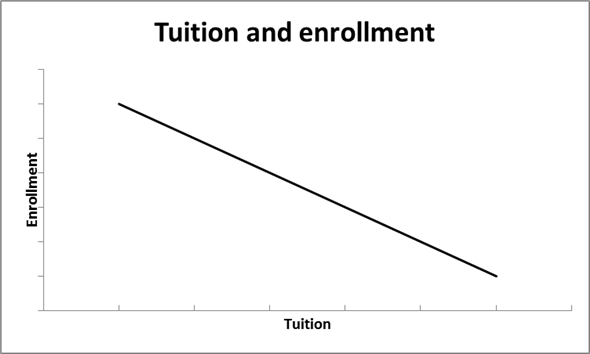(c) The more popular an entertainer is , the more likely tickets to her concerts will be in demand, and thus will cost a lot. Comparing this to an entertainer who is not well known, she would probably be happy by just getting people to come watch her even if she is not charging a lot for her concerts. So, we see here that the popularity of the entertainer and the price of her concert tickets exhibit a direct relationship, which is shown in the graph below.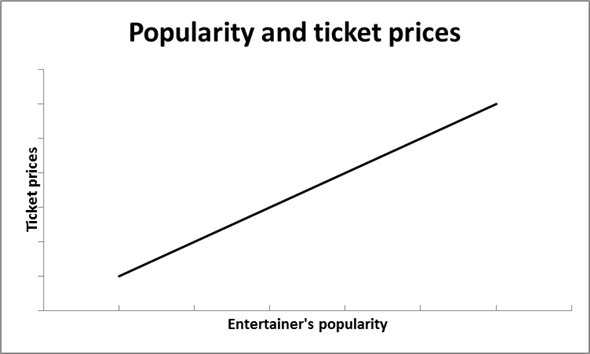Graphs are very important in economics as it represents the behavior of one variable when another variable moves. For example, a very important relation that is seen in introductory economics is the relationship between price and quantity. If we are looking at consumers, we would expect that as the price of goods goes up, the quantity demanded of the good will go down. The relation that as one variable goes up, the other variable goes down is called an inverse relationship, and can be represented with a downward sloping line as shown in the graph below.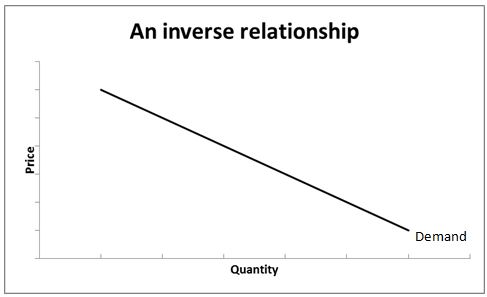A direct relationship between two variables can be defined in this way: if one variable goes up, the other variable also goes up. This is mostly seen in the supply of a good. If we are look at the producers of a good, we would expect that when the price is low, producers probably don't want to produce that much. However, when the price is high, producers can make more money and thus will want to sell more of that item. Thus, here, we will get a graph with an upward sloping line as shown below.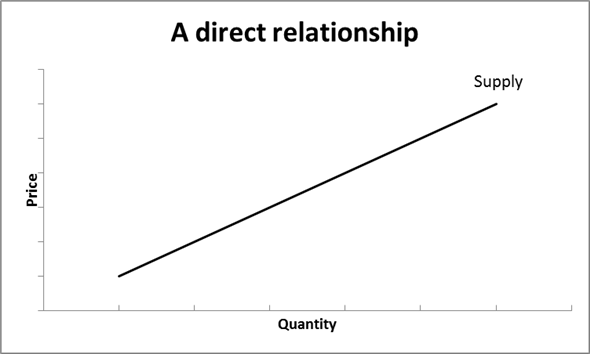If potatoes cost \$1.00 per pound, then the marginal cost of a pound of potatoes (which just means the additional cost to get one more pound of potatoes) is \$1.00. The marginal benefit (which just means the additional benefit or value that is received from having one more pound of potatoes) is the value that is placed on each pound of the potatoes - \$1.50, \$1.14, and \$1.05 for the first, second, and third pounds and \$0.30 for each pound after the third. It would make sense for you to buy the first pound of potatoes, since it cost \$1.00 and you value it at \$1.50. It will also make sense to get the second pound because you value it at \$1.14 and you can get it for \$1.00. Similarly, the third pound is also worth it because the value of \$1.05 you place on it is higher than the cost. However, it is not worth buying potatoes after the third pound. For example, the fourth pound will cost you \$1.00 as well, but you only value the fourth pound of potatoes at \$0.30. Your money is better spent on other goods that you find worth it! Let's check that we are within budget - adding the cost of the first, second, and third pounds of potatoes, we get \$3.69, which is within the \$5.00 budget. If you only had \$2.00, you would not be able to afford three pounds of potatoes, even though you still think it is a good deal (since the marginal benefit is higher than the marginal cost at the third pound of potatoes). You will still think it is worth it to buy the first (since you value the first pound at \$1.50 and it cost \$1.00) and the second (which you value at \$1.14 and cost \$1.00), so you will end up buying 2 pounds of potatoes with the \$2 you have.